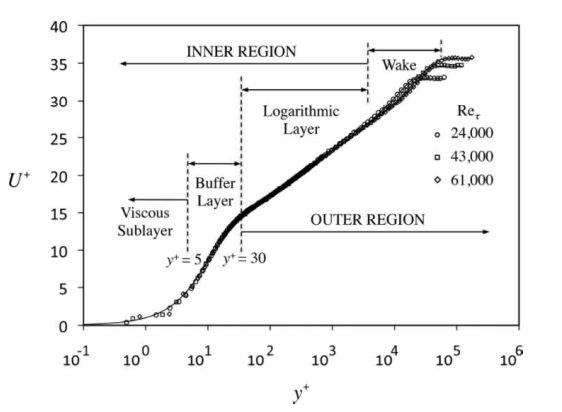WRITTEN BY:

This blog post is a follow up to a previous blog post (Part 1).  In the first blog post the following topics were discussed:

• What is a y+ value?
• Laminar vs turbulent flow.
• How to determine if your flow is laminar or turbulent?
• Introduction to wall functions.

In this blog we will go into more detail with regards to wall functions. The following topics will be discussed:

• What is a boundary layer?
• What is the viscous sublayer, the buff layer and the log-law layer?
• What is a wall function?
• What is y+ and U+ ?
• What ranges of y+ should I target?
• How do I target a specific y+ value?

The focus of this blog post is essentially to get a better understanding of what is going on in the graph plotted in Figure 1.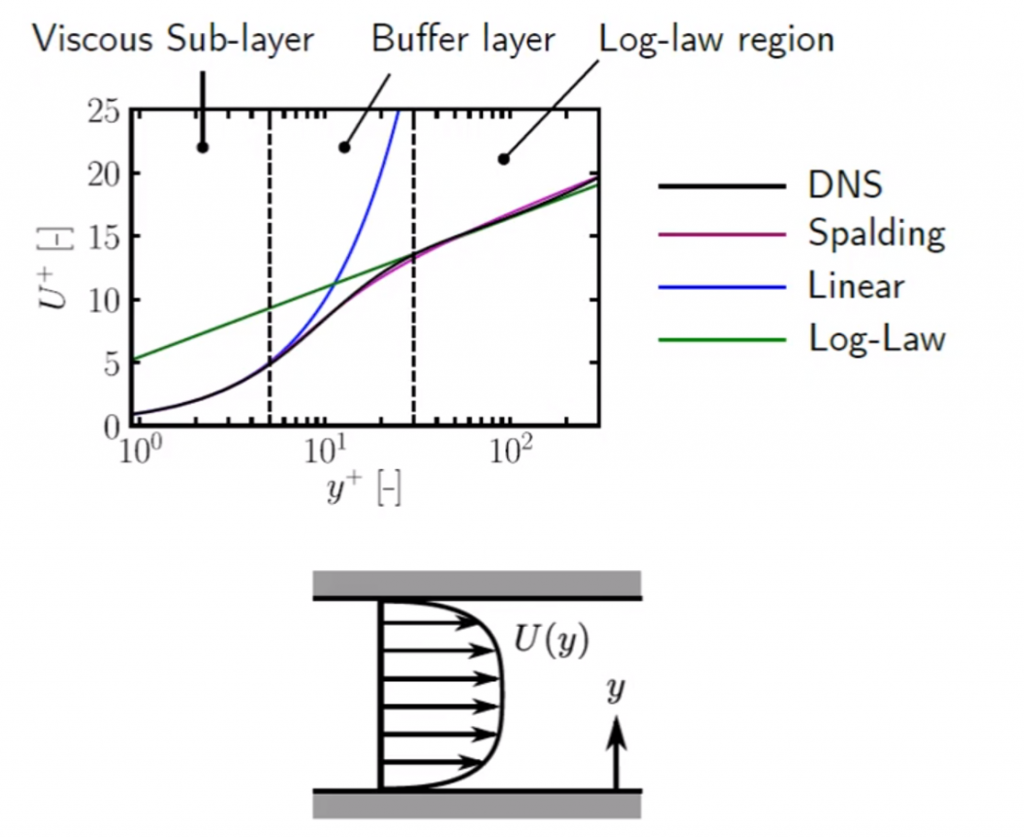Figure 1: Wall Functions

# What is a boundary layer?

A boundary layer is created anywhere where a moving fluid meets a no-slip wall. The boundary layer is created because the velocity at the no-slip wall is 0 m/s and it increases to the free stream velocity as it moves further away from the no-slip wall. The boundary layer and velocity profiles for the different flow types is illustrated in Figure 2.  For the purpose of this blog post we will specifically look at the turbulent boundary layer.

The boundary layer thickness is also an interesting topic as this is also something that needs to be considered when generating a mesh. This topic will however be covered in part 3.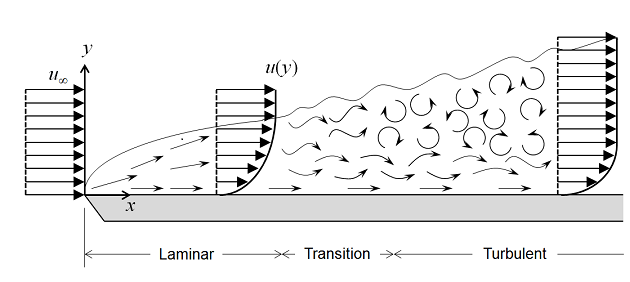Figure 2: Boundary Layer at No-Slip Wall

# What is the viscous sublayer, the buff layer and the log-law layer?

The turbulent boundary layer can be divided into 4 regions based on their distance from the no-slip wall as showed in Figure 3:

• Viscous sublayer
• Buff layer
• Log-law layer (Overlap region)
• Wake

The first 3 regions are known as the inner region (Flow is dominated by viscous effects) and the last 2 regions are known as the outer region. For the purpose of this blog post we are only interested in the inner region. An illustration of the velocity profile with the different boundary layer regions are showed in Figure 4.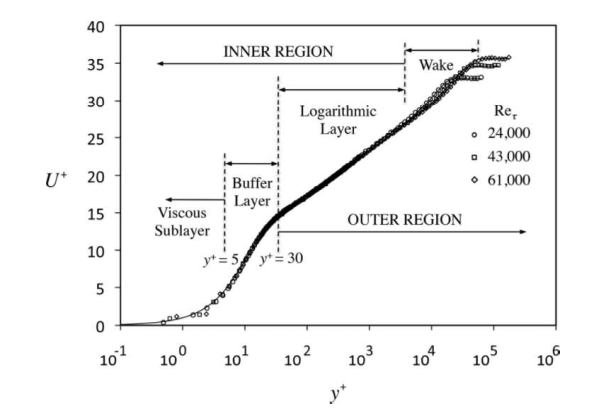Figure 3: Boundary Layer Regions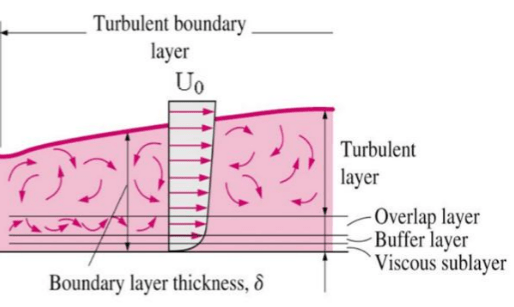Figure 4: Velocity Profile of Boundary Layer Regions

## Viscous sublayer

The viscous sublayer, also called the laminar sublayer, is a very thin layer near to a no-slip wall where the flow is dominated by viscous effects and the flow is laminar. The velocity profile in this layer can also be approximated to be linear and can also be seen in Figure 1 (Remember the  y+ values are given on a log scale).

## Buff layer

The buff layer is the layer where the turbulent effects are becoming significant but the flow is still dominated mainly by viscous effects.

## Log-law layer

The log-law layer, also called the overlap layer, is the layer where the turbulent effects are much more significant, but the flow is still not fully turbulent.

# What is a wall function?

A wall function describes the velocity as a function of distance from the wall.

We already know from the previous blog post that there are high velocity gradients close to the no-slip wall as the velocity increases from 0 m/s (at the wall) to the free stream velocity. We also know that in the region close to the no-slip wall we cannot use the normal moment conservation laws to describe the velocity profile and thus we need to use wall functions.

Wall functions are derived from empirical data that was measured from flow between 2 flat plates as showed in Figure 1. The DNS (Direct Numerical Simulation) fits the experimentally measured data almost exactly but this is an expensive analysis to do, so in most cases a curve is fitted to the empirical data. Two curves are usually fitted to the empirical data, a linear curve (Equation 1, blue curve in Figure 1), and log curve (Equation 2, green curve in Figure 1). A single polynomial curve can also be fitted to the empirical data. An example of a polynomial curve that fits the empirical data fairly accurately is known as the Spalding curve (Equation 3, purple curve in Figure 1).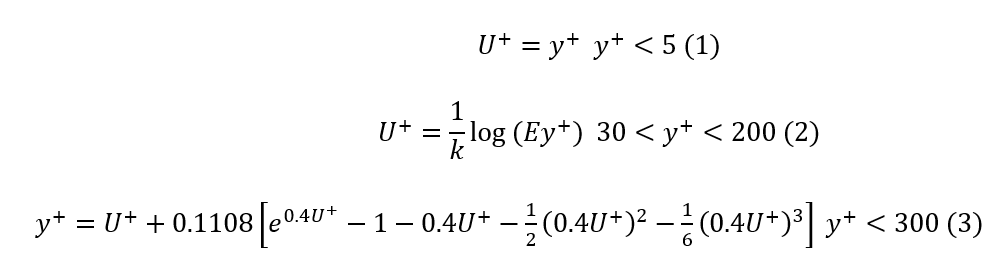• y+ : Dimensionless distance to the wall
• U+ : Dimensionless velocity
• k : Kaman constant
• E : Constant

Equation 1 or 2 can be used as is but it will give very inaccurate results for certain y+ ranges as can be seen in Figure 1, this is why in most cases Equation 1 and 2 are combined in CFD codes using a simple IF statement (Equation 4). Equation 3 is also implemented in some CFD codes but because you cannot isolate U+ in Equation 3 you would have to iterate to obtain a value for U+ . This can quickly become a very expensive computational exercise as the iteration process has to be performed at least once for every cell where the velocity needs to be calculated using a wall function per cycle.

It is due to the iterative posses that Equation 1 and 2 are used in combination with one another.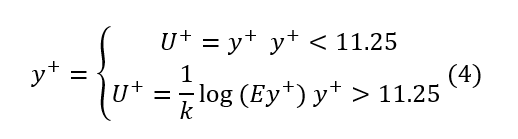It is clear from Figure 1 that using Equation 4 as is will yield inaccurate results in the buffer layer and that is why it is often recommend that the buffer layer is avoided when targeting a y+ value. In most cases CFD codes use some type of blending formulation of Equation 1 and 2 in the buffer layer but this differs between different CFD packages.

If the combination of Equation 1 and 2 are inaccurate and Equation 3 is computational expensive, why don’t we target a single y+ value in the viscous sublayer or in the log-law layer?

In general, this is the idea when targeting a specific y+ value but it is impossible to get all the fluid cells at the no-slip wall to a specific y+ value or even in a specific layer.  Why this is impossible will become clear at a later stage.

At this point we have explained most of what is showed in Figure 1 but you will probably ask what is y+ and U+ .

## What is y+ and U+ ?

To be able to understand y+ and U+ we need to look at how they are calculated. The equations to calculate y+ and U+ are given by Equations 5 and 6 respectively.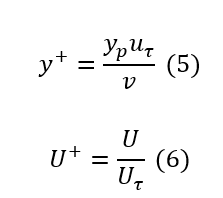• yp: Distance normal to the wall where y+ is calculated
• U: Velocity parallel to the wall as a function of y
• Uτ: Shear velocity
• v : Kinimatic viscosity

Some of the quantities given in Equations 5 and 6 you should be familiar with but you may have questions about some of them.

How is Uτ calculated?

The shear velocity is calculated from the shear stress at the no-slip wall as given by Equation 7 and the shear stress is calculated using Equation 8.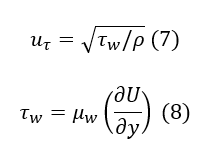• yp: Wall shear stress
• ρ: Fluid density
• μw : Dynamic wall viscosity
• y: Distance from wall

Some CFD codes use the turbulent kinetic energy to calculate the shear velocity and then y+ is given as y*. The difference between y+ and y* and how the shear stress is calculated will be discussed in another blog post.

How is yp  calculated?

yp is usually the distance from the wall to the cell centroid directly adjacent to the wall as illustrated in Figure 5.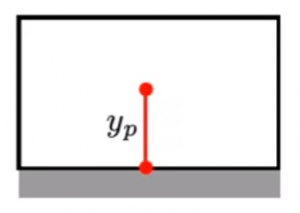Figure 5: y_p Distance

This means that your cell high adjacent to the wall should be H=2yp and this is what you use as a starting point to decide how fine or course your mesh should be in the region of the boundary wall.

# What ranges of y+ should I target?

We have now seen different wall functions that is valid for different y+ values. In general, we try to target one of the following y+ ranges: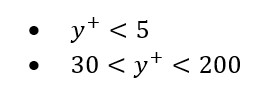but again, this will be dependent on the CFD code and wall functions being used.

Another factor that influences the targeted y+ value is the turbulent model that is being used.  In some cases the terms low (Targeted y+ is in the viscous sublayer) and high (Targeted y+ is in the log-law layer) Reynolds number are used to indicate whether the model uses the linear wall function or the log-law function. We know that a combination of the linear and log-law functions are used as it is not possible to get all your y+ values in a specific region. It is important to know that when a high Reynold number model is used, a low Reynolds number wall function is used where the y+<11.25 but the low Reynolds number effects will not be considered. This is not necessarily how it is always implemented and it is important to consult the reference manual of the CFD code being used.

In general the velocity profile that will be approximated in the fluid cell adjacent to the no-slip wall will look like the illustrations in Figure 6 for the low and Figure 7 for the high Reynolds number turbulence models.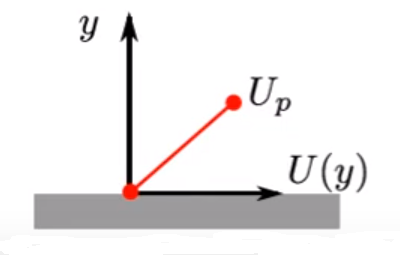Figure 6: Velocity Approximation in First Cell Adjacent to No-Slip Wall (Low-Reynolds Number)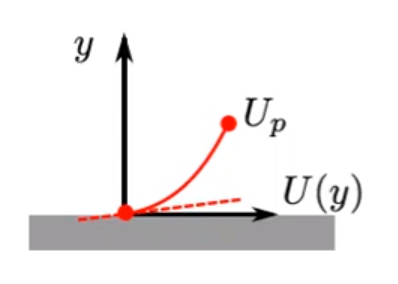Figure 7: Velocity Approximation in First Cell Adjacent to No-Slip Wall (High-Reynolds Number)

At this stage you should have a good idea of what is going on in Figure 1 and what ranges of y+ you should target. The question now is how do I calculate my cell size to target a specific y+ value and how do I calculate the shear stress?

Luckily there is online calculators available to approximate an initial guess of yp. This is just an initial guess and usually a couple of iterations is required to target a specific y+ value in the regions of interest.

I would recommend starting at Dr Aidan Wimshurst website where an online calculator and an explanation of how the calculator works is given.

This will be the last blog post for 2019 but in Part 3 next year we will put all of the topics discussed thus far in to practice using the Cradle scFlow software.